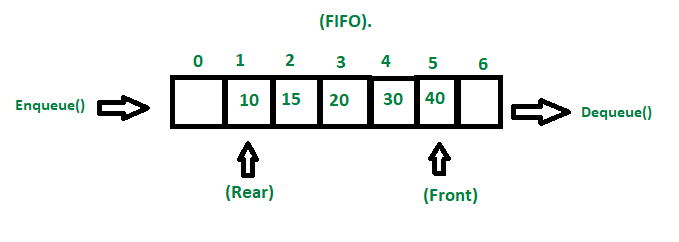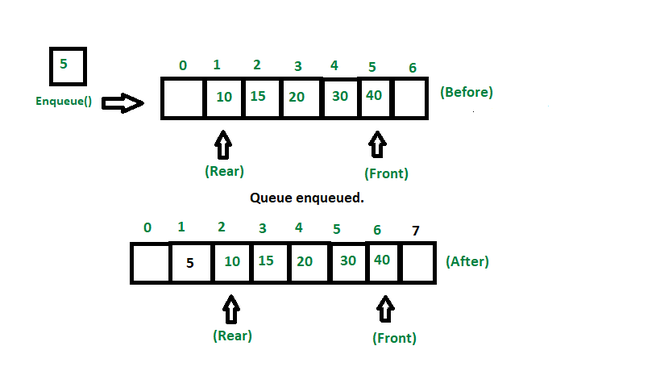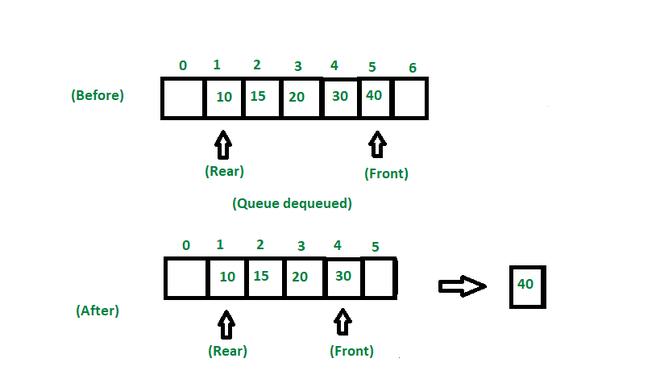# Introduction to Queue in Data Structures and Algorithms

• Difficulty Level : Expert
• Last Updated : 05 Aug, 2022

## What is Queue?

A queue is a linear data structure that is open at both ends and the operations are performed in First In First Out (FIFO) order.

We define a queue to be a list in which all additions to the list are made at one end, and all deletions from the list are made at the other end.  The element which is first pushed into the order, the operation is first performed on that.

## FIFO Principle of Queue:

• A Queue is like a line waiting to purchase tickets, where the first person in line is the first person served. (i.e. First come first serve).
• Position of the entry in a queue ready to be served, that is, the first entry that will be removed from the queue, is called the front of the queue(sometimes, head of the queue), similarly, the position of the last entry in the queue, that is, the one most recently added, is called the rear (or the tail) of the queue. See the below figure.FIFO property of queue

## Characteristics of Queue:

• Queue can handle multiple data.
• We can access both ends.
• They are fast and flexible.

## Queue Representation:

### Array Representation:

Like stacks, Queues can also be represented in an array: In this representation, the Queue is implemented using the array. Variables used in this case are

• Queue: the name of the array storing queue elements.
• Front: the index where the first element is stored in the array representing the queue.
• Rear: the index where the last element is stored in an array representing the queue.

Array representation of queue:

## C++

 `// Creating an empty queue ` ` `  `#include ` `using` `namespace` `std; ` ` `  `// A structure to represent a queue ` `class` `Queue { ` `public``: ` `    ``int` `front, rear, size; ` `    ``unsigned cap; ` `    ``int``* arr; ` `}; ` ` `  `// Function to create a queue of given capacity ` `// It initializes size of queue as 0 ` `Queue* createQueue(unsigned cap) ` `{ ` `    ``Queue* queue = ``new` `Queue(); ` `    ``queue->cap = cap; ` `    ``queue->front = queue->size = 0; ` ` `  `    ``queue->rear = cap - 1; ` `    ``queue->arr = ``new` `int``[(queue->cap * ``sizeof``(``int``))]; ` `    ``return` `queue; ` `}`

A queue can also be represented in Linked-lists, Pointers, and Structures.

## C++

 `struct` `QNode { ` `    ``int` `data; ` `    ``QNode* next; ` `    ``QNode(``int` `d) ` `    ``{ ` `        ``data = d; ` `        ``next = NULL; ` `    ``} ` `}; ` ` `  `struct` `Queue { ` `    ``QNode *front, *rear; ` `    ``Queue() ` `    ``{ ` `        ``front = rear = NULL; ` `    ``} ` `};`

## Types of Queue:

There are different types of queue:

### Input Restricted Queue

This is a simple queue. In this type of queue, the input can be taken from only one end but deletion can be done from any of the ends.

### Output Restricted Queue

This is also a simple queue. In this type of queue, the input can be taken from both ends but deletion can be done from only one end.

### Circular Queue

This is a special type of queue where the last position is connected back to the first position. Here also the operations are performed in FIFO order.

### Double-Ended Queue (Deque)

In a double-ended queue the insertion and deletion operations, both can be performed from both ends.

### Priority Queue

A priority queue is a special queue where the elements are accessed based on the priority assigned to them.

## Basic Operations of Queue:

There are few supporting operations (auxiliary operations):

### front():

This operation returns the element at the front end without removing it.

## C++

 `// Function to get front of queue ` `int` `front(Queue* queue) ` `{ ` `    ``if` `(isempty(queue)) ` `        ``return` `INT_MIN; ` `    ``return` `queue->arr[queue->front]; ` `}`

### rear():

This operation returns the element at the rear end without removing it.

## C++

 `// Function to get rear of queue ` `int` `rear(Queue* queue) ` `{ ` `    ``if` `(isempty(queue)) ` `        ``return` `INT_MIN; ` `    ``return` `queue->arr[queue->rear]; ` `}`

### isEmpty():

This operation returns a boolean value that indicates whether the queue is empty or not.

## C++

 `// This function will check weather ` `// the queue is empty or not: ` `boolisEmpty() ` `{ ` `    ``if` `(front == -1) ` `        ``return` `true``; ` `    ``else` `        ``return` `false``; ` `}`

### isFull():

This operation returns a boolean value that indicates whether the queue is full or not.

## C++

 `// This function will check ` `// weather the queue is full or not. ` `boolisFull() ` `{ ` `    ``if` `(front == 0 && rear == MAX_SIZE - 1) { ` `        ``return` `true``; ` `    ``} ` `    ``return` `false``; ` `}`

### Enqueue():

Adds (or stores) an element to the end of the queue.

The following steps should be taken to enqueue (insert) data into a queue:

• Step 1: Check if the queue is full.
• Step 2: If the queue is full, return overflow error and exit.
• Step 3: If the queue is not full, increment the rear pointer to point to the next empty space.
• Step 4: Add the data element to the queue location, where the rear is pointing.
• Step 5: return success.Enqueue representation

Implementation of Enqueue:

## C++

 `void` `queueEnqueue(``int` `data) ` `{ ` `    ``// Check queue is full or not ` `    ``if` `(capacity == rear) { ` `        ``printf``(``"\nQueue is full\n"``); ` `        ``return``; ` `    ``} ` ` `  `    ``// Insert element at the rear ` `    ``else` `{ ` `        ``queue[rear] = data; ` `        ``rear++; ` `    ``} ` `    ``return``; ` `}`

### Dequeue():

Removes (or access) the first element from the queue.

The following steps are taken to perform the dequeue operation:

• Step 1: Check if the queue is empty.
• Step 2: If the queue is empty, return the underflow error and exit.
• Step 3: If the queue is not empty, access the data where the front is pointing.
• Step 4: Increment the front pointer to point to the next available data element.
• Step 5: The Return success.Dequeue operation

Implementation of dequeue:

## C++

 `void` `queueDequeue() ` `{ ` `    ``// If queue is empty ` `    ``if` `(front == rear) { ` `        ``printf``(``"\nQueue is empty\n"``); ` `        ``return``; ` `    ``} ` ` `  `    ``// Shift all the elements from index 2 ` `    ``// till rear to the left by one ` `    ``else` `{ ` `        ``for` `(``int` `i = 0; i < rear - 1; i++) { ` `            ``queue[i] = queue[i + 1]; ` `        ``} ` ` `  `        ``// decrement rear ` `        ``rear--; ` `    ``} ` `    ``return``; ` `}`

## Implementation of queue:

Below is an implementation of all the operations used in a queue.

## C++

 `// Implementation of queue(enqueue, dequeue). ` `#include ` `using` `namespace` `std; ` ` `  `class` `Queue { ` `public``: ` `    ``int` `front, rear, size; ` `    ``unsigned cap; ` `    ``int``* arr; ` `}; ` ` `  `Queue* createQueue(unsigned cap) ` `{ ` `    ``Queue* queue = ``new` `Queue(); ` `    ``queue->cap = cap; ` `    ``queue->front = queue->size = 0; ` ` `  `    ``queue->rear = cap - 1; ` `    ``queue->arr = ``new` `int``[(queue->cap * ``sizeof``(``int``))]; ` `    ``return` `queue; ` `} ` ` `  `int` `isFull(Queue* queue) ` `{ ` `    ``return` `(queue->size == queue->cap); ` `} ` ` `  `int` `isempty(Queue* queue) { ``return` `(queue->size == 0); } ` `// Function to add an item to the queue. ` `// It changes rear and size. ` `void` `enqueue(Queue* queue, ``int` `item) ` `{ ` `    ``if` `(isFull(queue)) ` `        ``return``; ` `    ``queue->rear = (queue->rear + 1) % queue->cap; ` `    ``queue->arr[queue->rear] = item; ` `    ``queue->size = queue->size + 1; ` `    ``cout << item << ``" enqueued to queue\n"``; ` `} ` `// Function to remove an item from queue. ` `// It changes front and size ` `int` `dequeue(Queue* queue) ` `{ ` `    ``if` `(isempty(queue)) ` `        ``return` `INT_MIN; ` `    ``int` `item = queue->arr[queue->front]; ` `    ``queue->front = (queue->front + 1) % queue->cap; ` `    ``queue->size = queue->size - 1; ` `    ``return` `item; ` `} ` `int` `front(Queue* queue) ` `{ ` `    ``if` `(isempty(queue)) ` `        ``return` `INT_MIN; ` `    ``return` `queue->arr[queue->front]; ` `} ` `int` `rear(Queue* queue) ` `{ ` `    ``if` `(isempty(queue)) ` `        ``return` `INT_MIN; ` `    ``return` `queue->arr[queue->rear]; ` `} ` ` `  `// Driver code ` `int` `main() ` `{ ` `    ``Queue* queue = createQueue(1000); ` `    ``enqueue(queue, 10); ` `    ``enqueue(queue, 20); ` `    ``enqueue(queue, 30); ` `    ``enqueue(queue, 40); ` `    ``cout << dequeue(queue); ` `    ``cout << ``" dequeued from queue\n"``; ` `    ``cout << ``"Front item is "` `<< front(queue) << endl; ` `    ``cout << ``"Rear item is "` `<< rear(queue); ` `    ``return` `0; ` `}`

Output

```10 enqueued to queue
20 enqueued to queue
30 enqueued to queue
40 enqueued to queue
10 dequeued from queue
Front item is 20
Rear item is 40```

Time complexity: All the operations have O(1) time complexity.
Auxiliary Space: O(N)

## Applications of Queue:

Application of queue is common. In a computer system, there may be queues of tasks waiting for the printer, for access to disk storage, or even in a time-sharing system, for use of the CPU. Within a single program, there may be multiple requests to be kept in a queue, or one task may create other tasks, which must be done in turn by keeping them in a queue.

• It has a single resource and multiple consumers.
• It synchronizes between slow and fast devices.
• In a network, a queue is used in devices such as a router/switch and mail queue.
• Variations: dequeue, priority queue and double-ended priority queue.

### 1. What data structure can be used to implement a priority queue?

Priority queues can be implemented using a variety of data structures, including linked lists, arrays, binary search trees, and heaps. Priority queues are best implemented using the heap data structure.

### 2. Queues are used for what purpose?

In addition to making your data persistent, queues reduce errors that occur when different parts of your system are down.

### 3. In data structures, what is a double-ended queue?

In a double-ended queue, elements can be inserted and removed at both ends.

### 4. What is better, a stack or a queue?

If you want things to come out in the order you put them in, use a queue. Stacks are useful when you want to reorder things after putting them in.

Related articles:

My Personal Notes arrow_drop_up
Recommended Articles
Page :